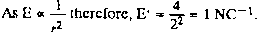# The electric field due to a positive charge at a distance 1 m is 4 NC–1. Its magnitude at distance 2 m will be    (A)    0.5 NC–1    (B)    1 NC–1    (C)    2 NC–1    (D)    none of the above9 years ago9 years ago

E1=4 N/C,

r1=1,

therefore,E1=(k x q )/r1^2

q=E1/k ----------(1)

Given r2=2

E2=(k x q)/r2^2 [By substituting (1)]

E2=E1/4

E2=1

Ans:(B)